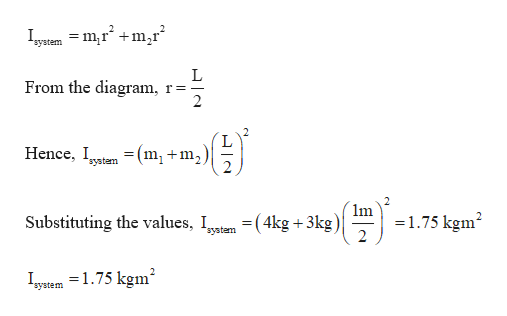Question
1 views

A light rod of length l= 1.00 m
to its length and passing
through its center as in
Figure P8.51. Two particles of
masses m1 = 4.00 kg and m2 =3.00 kg are connected to the
ends of the rod. (a) Neglecting
the mass of the rod, what is the
system’s kinetic energy when
its angular speed is 2.50 rad/s?
(b) Repeat the problem, assuming
the mass of the rod is taken
to be 2.00 kg.

check_circle

Step 1

Given:

m1 = 4.00 kg

m2 =3.00 kg

Length of the rod, L = 1 m

Angular velocity of rod = 2.5 rad/s

Step 2

Calculating the moment of inertia of the system given that rod is of negligible mass:help_outlineImage TranscriptioncloseIystam = m,r +m,r From the diagram, r== 2 Hence, Iatem = (m, +m, system 1m =1.75 kgm? Substituting the values, Iatam = (4kg +3kg) system Iyuem =1.75 kgm² וב fullscreen
Step 3

Calculating the kinetic en...

### Want to see the full answer?

See Solution

#### Want to see this answer and more?

Solutions are written by subject experts who are available 24/7. Questions are typically answered within 1 hour.*

See Solution
*Response times may vary by subject and question.
Tagged in

### Angular Motion